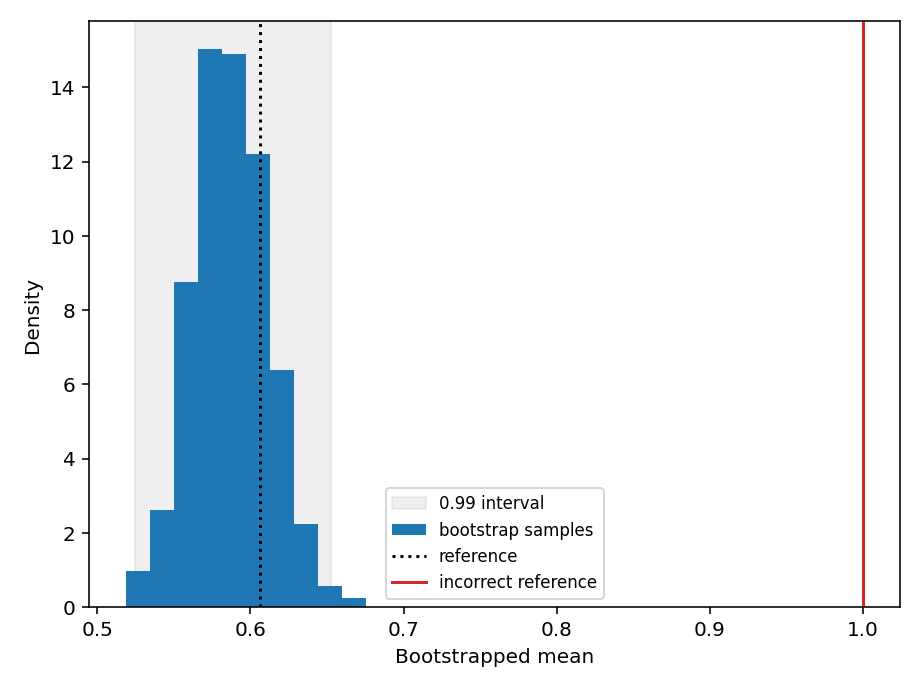# 🧪 pytest-bootstrap¶Scientific software development often relies on stochasticity, e.g. for Monte Carlo integration or simulating the Ising model. Testing non-deterministic code is difficult. This package offers a bootstrap test to validate stochastic algorithms, including multiple hypothesis correction for vector statistics. It can be installed by running pip install pytest-bootstrap.

## Example¶

Suppose we want to implement the expected value of log-normal distribution with location parameter $$\mu$$ and scale parameter $$\sigma$$.

>>> import numpy as np
>>>
>>> def lognormal_expectation(mu, sigma):
...   return np.exp(mu + sigma ** 2 / 2)
>>>
>>> def lognormal_expectation_wrong(mu, sigma):
...   return np.exp(mu + sigma ** 2)


We can validate our implementation by simulating from a lognormal distribution and comparing with the bootstrapped mean.

>>> from pytest_bootstrap import bootstrap_test
>>>
>>> mu = -1
>>> sigma = 1
>>> reference = lognormal_expectation(mu, sigma)
>>> x = np.exp(np.random.normal(mu, sigma, 1000))
>>> result = bootstrap_test(x, np.mean, reference)


This returns a summary of the test, such as the bootstrapped statistics.

>>> result.keys()
dict_keys(['alpha', 'alpha_corrected', 'reference', 'lower', 'upper',
'z_score', 'median', 'iqr', 'tol', 'statistics'])


Comparing with our incorrect implementation reveals the bug.

>>> reference_wrong = lognormal_expectation_wrong(mu, sigma)
>>> result = bootstrap_test(x, np.mean, reference_wrong)
Traceback (most recent call last):
...
pytest_bootstrap.BootstrapTestError: the reference value 1.0 lies outside
the 1 - (alpha = 0.01) interval ...


Visualising the bootstrapped distribution using pytest_bootstrap.result_hist() can help identify discrepancies between the bootstrapped statistics and the theoretical reference value. Note that you need to install matplotlib separately or install pytest-bootstrap using pip install pytest-bootstrap[plot].Histogram of bootstrapped means reveals the erroneous implementation of the log-normal mean.¶

A comprehensive set of examples can be found in the tests.

## Interface¶

exception pytest_bootstrap.BootstrapTestError(result: dict)

Reference value falls outside bootstrapped confidence interval.

pytest_bootstrap.bootstrap_test(samples: numpy.ndarray, statistic: Callable, reference: float, statistic_args: = None, statistic_kwargs: = None, num_bootstrap_samples: int = 1000, alpha: float = 0.01, multiple_hypothesis_correction: str = 'bonferroni', rtol: float = 1e-07, atol: float = 0, on_fail: str = 'raise') dict

Compare a bootstrap sample of a statistic evaluated on i.i.d samples from a stochastic process with a reference value.

The test will fail if the reference lies outside the 1 - alpha posterior interval, i.e. is smaller than the empirical alpha / 2 quantile or larger than the 1 - alpha / 2 quantile. The relative rtol and absolute tolerance atol are added together to obtain an overall tolerance atol + rtol * abs(reference) for the interval test (see numpy.isclose() for further details).

Parameters
• samples – I.i.d. samples from a stochastic process on which to evaluate the statistic.

• statistic – Function to evaluate a statistic on a bootstrapped sample.

• reference – Reference value to compare with the bootstrap sample of the statistic.

• statistic_args – Positional arguments forwarded to the statistic.

• statistic_kwargs – Keyword arguments forwarded to the statistic.

• num_bootstrap_samples – Number of bootstrap samples.

• alpha – Significance level to use for testing agreement between the bootstrapped distribution of the statistic and the reference value. alpha roughly corresponds to the probability that the test will fail even if the reference value is correct. This value should be small for the test to pass with high probability.

• multiple_hypothesis_correction – Method used to correct for multiple hypotheses being tested if the statistic is vector-valued. False disables multiple hypothesis correction.

• rtol – Relative tolerance.

• atol – Absolute tolerance.

• on_fail – Raise a BootstrapTestError if raise or warn if warn.

Returns

result – Dictionary of test information, comprising

• alpha_corrected – Significance level after multiple hypothesis correction. Equal to alpha if the statistic is a scalar or no multiple hypothesis correction is applied.

• lower – Lower limit of the 1 - alpha bootstrapped interval, i.e. the alpha / 2 quantile.

• upper – Upper limit of the 1 - alpha bootstrapped interval, i.e. the 1 - alpha / 2 quantile.

• iqr – Interquartile range of the bootstrapped statistic.

• median – Median of the bootstrapped statistic.

• z_score – Z-score of the reference value under the bootstrapped distribution, i.e. (reference - mean(s)) / std(s), where s is the vector of bootstrapped statistic.

pytest_bootstrap.result_hist(result: dict, show_interval: bool = True, ax=None, **kwargs) None

Plot a histogram of bootstrapped statistics together with the reference value and $$1 - \alpha$$ interval.

Note

Use on_fail = 'warn' as an argument to bootstrap_test() to obtain test results for a failing test.

Parameters
• result – Bootstrap test result generated by bootstrap_test().

• show_interval – Whether to show the $$1 - \alpha$$ interval.

• ax – Axes to use for plotting